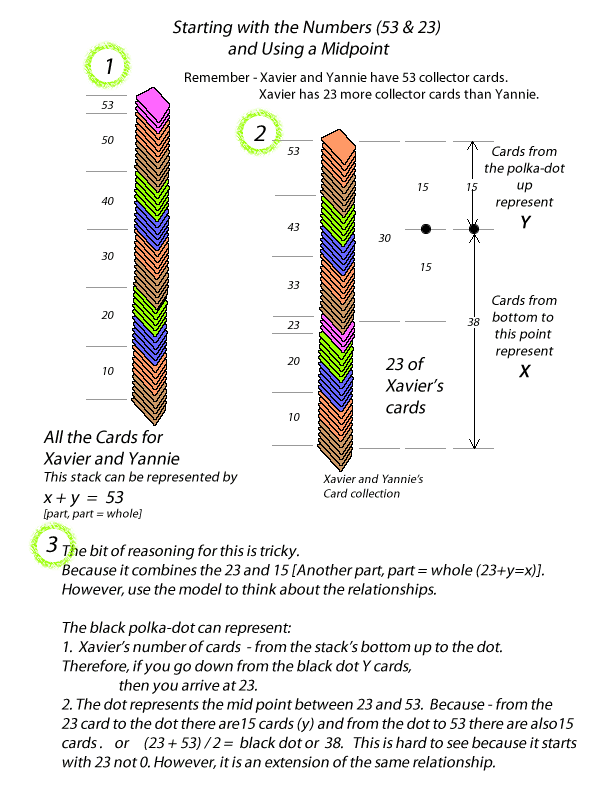# Simultaneous Equations

### Solving equations with substitution - Formal operational, Systematic, Someone with a lot of algebra.### Concrete operationallyThis bit of information is crucial for people to better understand equality and use it to represent ideas mathematically and for solving problems. Too often the only assiciation people have with equality is the equal sign with the meaning of solve or find the one number that is equal. This usually limits understanding of mathematics to calculation.

Push it FurtherIf you hung in to here. Congratulations! You're thinking like a mathematician.

Dr. Robert Sweetland's Notes ©